# Criterion for boundedness of power series

 Importance: Low ✭
 Author(s): Rüdinger, Andreas
 Subject: Analysis
 Keywords: boundedness power series real analysis
 Recomm. for undergrads: yes
 Posted by: andreasruedinger on: May 9th, 2009
Question   Give a necessary and sufficient criterion for the sequence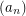so that the power series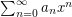is bounded for all.

Consider a power seriesthat is convergent for all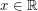, thus defining a function. Are there criteria to decide whetheris bounded (which e.g. is the case for the series with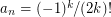forand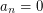for n odd)? Some general remarks:

\item A necessary condition forto be bounded is that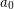is the only non-zero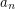or there are infinitely many non-zero's which change sign infinitely many times. \item Changing a finite set of's (except) does leave the subspace of bounded power series. \item The subspace of bounded power series is "large" in the sense that it is both a linear subspace (closed under sums and scalar multiples) and an algebra (closed under products). It includes all functions of the form, whereis any entire function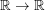. The question whether the subspace of bounded power series contains only these functions seems to be open.

### A necessary condition

It seems the sum would be bounded if there are only finitely many non-zero a sub n; it is not apparent to me that a sub 0 be the only non-zero a sub n.

### What you have then is a

What you have then is a polynomial, and any nonconstant polynomial function is unbounded.

### Re: A necessary condition

I posted the above comment anonymously, but now I have created an account. "It seems the sum would be bounded if there are only finitely many non-zero a sub n; it is not apparent to me that a sub 0 be the only non-zero a sub n."

### harder than that

Look at sin(x)=x-x^3/6+x^5/120-....

JPB

### sin x = cos(pi/2 - x)

The sine function is in the class mentioned.

## Comment viewing options

Select your preferred way to display the comments and click "Save settings" to activate your changes.